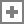# 略微加速

## PHP官方手册 - 互联网笔记

PHP - Manual: AppendIterator (官方文档)

2020-09-23

# The AppendIterator class

(PHP 5 >= 5.1.0, PHP 7)

## 类摘要

AppendIterator extends IteratorIterator implements OuterIterator {
/* 方法 */
public __construct ( void )
public append ( Iterator `\$iterator` ) : void
public current ( void ) : mixed
public getArrayIterator ( void ) : ArrayIterator
public getInnerIterator ( void ) : Iterator
public getIteratorIndex ( void ) : int
public key ( void ) : scalar
public next ( void ) : void
public rewind ( void ) : void
public valid ( void ) : bool
/* 继承的方法 */
public IteratorIterator::__construct ( Traversable `\$iterator` )
public IteratorIterator::current ( void ) : mixed
public IteratorIterator::key ( void ) : mixed
public IteratorIterator::next ( void ) : void
public IteratorIterator::rewind ( void ) : void
public IteratorIterator::valid ( void ) : bool
}add a note

### User Contributed Notes 5 notes

joshdifabio at gmail dot com
2 years ago
``` Note that AppendIterator will segfault when iterating over an empty generator. Do not use AppendIterator in conjunction with generators.https://3v4l.org/YC68khttps://bugs.php.net/bug.php?id=71436 ```
-1
frode at ennerd dot com
9 months ago
``` In many cases, especially for streaming sources, Generators are way more efficient. I noticed that the AppendIterator buffers the entire "inner iterator".<?php/** * This appends \$next iterator to \$iterator. */function append_iterators(...\$iterators){    foreach(\$iterators as \$iterator)        foreach(\$iterator as \$row)            yield(\$row);}/** * Merge iterator takes one first from each iterator until * every iterator is empty. */function merge_iterators(....\$its) {    \$numberOfIts = sizeof(\$its);    while(\$numberOfIts > 0) {        \$iterator = array_shift(\$its);        yield(\$iterator->current());        \$iterator->next();        if(\$iterator->valid())            \$its[] = \$iterator;        else            \$numberOfIts--;    }});?> ```
-1
koambarun at evolution dot com
2 years ago
``` \$append_iterator = new \AppendIterator();\$generator = ReportModel::come_generator();foreach (\$errors as \$value) {    //If first \$value not empty, generator is not empty.    if(!empty(\$value))    {        \$append_iterator->append(\$errors);        //break out of loop after appending.    break;    }} ```
-4
komalbarun at gmail dot com
2 years ago
``` Updated code. I could not find how to edit a note :/Preventing segfault if empty generator.<?php\$append_iterator = new \AppendIterator();\$generator = ReportModel::come_generator();// Only works if first value in generator is not empty// useful when yielding arraysforeach (\$append_iterator as \$value) {    //If first \$value not empty, generator is not empty.    if(!empty(\$value))    {        \$append_iterator->append(\$errors);        //break out of loop after appending.    break;    }} ```
-5
komalbarun at gmail dot com
2 years ago
``` Updated code. I could not find how to edit a note :/Preventing segfault if empty generator.<?php\$append_iterator = new \AppendIterator();\$generator = some_generator();// Only works if first value in generator is not empty// useful when yielding arraysforeach (\$generator as \$value) {    //If first \$value not empty, generator is not empty.    if(!empty(\$value))    {        \$append_iterator->append(\$generator );        //break out of loop after appending.        break;    }} ```